ctc 订阅
CTC

CTC
Adds "Close Tab" to the context menu, shown whenever Back, Forward, Stop and Reload... 已更新 2007 年 03 月 5 日

• 目前结合神经网络的端到端的声学模型训练方法主要CTC和基于Attention两种。 本文主要介绍CTC算法的基本概念，可能应用的领域，以及在结合神经网络进行CTC算法的计算细节。 CTC算法概念 CTC算法全称叫：...
目前主流的语音识别都大致分为特征提取，声学模型，语音模型几个部分。目前结合神经网络的端到端的声学模型训练方法主要CTC和基于Attention两种。

本文主要介绍CTC算法的基本概念，可能应用的领域，以及在结合神经网络进行CTC算法的计算细节。

CTC算法概念

CTC算法全称叫：Connectionist temporal classification。从字面上理解它是用来解决时序类数据的分类问题。

传统的语音识别的声学模型训练，对于每一帧的数据，需要知道对应的label才能进行有效的训练，在训练数据之前需要做语音对齐的预处理。而语音对齐的过程本身就需要进行反复多次的迭代，来确保对齐更准确，这本身就是一个比较耗时的工作。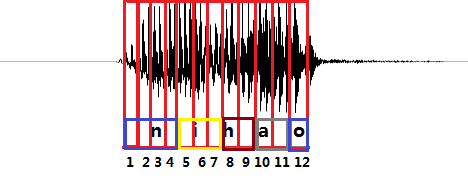图1 “你好”发音的波形示意图

如图1所示，是“你好”这句话的声音的波形示意图， 每个红色的框代表一帧数据，传统的方法需要知道每一帧的数据是对应哪个发音音素。比如第1,2,3,4帧对应n的发音，第5,6,7帧对应i的音素，第8,9帧对应h的音素，第10,11帧对应a的音素，第12帧对应o的音素。（这里暂且将每个字母作为一个发音音素）

与传统的声学模型训练相比，采用CTC作为损失函数的声学模型训练，是一种完全端到端的声学模型训练，不需要预先对数据做对齐，只需要一个输入序列和一个输出序列即可以训练。这样就不需要对数据对齐和一一标注，并且CTC直接输出序列预测的概率，不需要外部的后处理。

既然CTC的方法是关心一个输入序列到一个输出序列的结果，那么它只会关心预测输出的序列是否和真实的序列是否接近（相同），而不会关心预测输出序列中每个结果在时间点上是否和输入的序列正好对齐。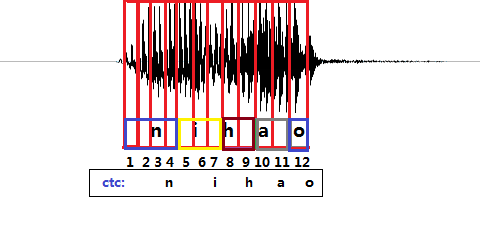图2 ctc预测结果示意图

CTC引入了blank（该帧没有预测值），每个预测的分类对应的一整段语音中的一个spike（尖峰），其他不是尖峰的位置认为是blank。对于一段语音，CTC最后的输出是spike（尖峰）的序列，并不关心每一个音素持续了多长时间。
如图2所示，拿前面的nihao的发音为例，进过CTC预测的序列结果在时间上可能会稍微延迟于真实发音对应的时间点，其他时间点都会被标记会blank。
这种神经网络+CTC的结构除了可以应用到语音识别的声学模型训练上以外，也可以用到任何一个输入序列到一个输出序列的训练上（要求：输入序列的长度大于输出序列）。
比如，OCR识别也可以采用RNN+CTC的模型来做，将包含文字的图片每一列的数据作为一个序列输入给RNN+CTC模型，输出是对应的汉字，因为要好多列才组成一个汉字，所以输入的序列的长度远大于输出序列的长度。而且这种实现方式的OCR识别，也不需要事先准确的检测到文字的位置，只要这个序列中包含这些文字就好了。

RNN+CTC模型的训练

下面介绍在语音识别中，RNN+CTC模型的训练详细过程，到底RNN+CTC是如何不用事先对齐数据来训练序列数据的。
首先，CTC是一种损失函数，它用来衡量输入的序列数据经过神经网络之后，和真实的输出相差有多少。

比如输入一个200帧的音频数据，真实的输出是长度为5的结果。 经过神经网络处理之后，出来的还是序列长度是200的数据。比如有两个人都说了一句nihao这句话，他们的真实输出结果都是nihao这5个有序的音素，但是因为每个人的发音特点不一样，比如，有的人说的快有的人说的慢，原始的音频数据在经过神经网络计算之后，第一个人得到的结果可能是：nnnniiiiii…hhhhhaaaaaooo(长度是200)，第二个人说的话得到的结果可能是：niiiiii…hhhhhaaaaaooo(长度是200)。这两种结果都是属于正确的计算结果，可以想象，长度为200的数据，最后可以对应上nihao这个发音顺序的结果是非常多的。CTC就是用在这种序列有多种可能性的情况下，计算和最后真实序列值的损失值的方法。

详细描述如下：

训练集合为S={(x1,z1),(x2,z2),...(xN,zN)}$S=\left\{\left({x}^{1},{z}^{1}\right),\left({x}^{2},{z}^{2}\right),...\left({x}^{N},{z}^{N}\right)\right\}$$S=\lbrace (x^1,z^1), (x^2, z^2), ...(x^N,z^N) \rbrace$, 表示有N$N$$N$个训练样本，x$x$$x$是输入样本，z$z$$z$是对应的真实输出的label。一个样本的输入是一个序列，输出的label也是一个序列，输入的序列长度大于输出的序列长度。

对于其中一个样本(x,z)$\left(x,z\right)$$(x,z)$，x=(x1,x2,x3,...,xT)$x=\left({x}_{1},{x}_{2},{x}_{3},...,{x}_{T}\right)$$x=(x_1,x_2,x_3,...,x_T)$表示一个长度为T帧的数据，每一帧的数据是一个维度为m的向量，即每个xi∈Rm${x}_{i}\in {R}^{m}$$x_i \in R^m$。 xi${x}_{i}$$x_i$可以理解为对于一段语音，每25ms作为一帧，其中第i$i$$i$帧的数据经过MFCC计算后得到的结果。

z=(z1,z2,z3,...zU)$z=\left({z}_{1},{z}_{2},{z}_{3},...{z}_{U}\right)$$z=(z_1,z_2, z_3,...z_U)$表示这段样本语音对应的正确的音素。比如，一段发音“你好”的声音，经过MFCC计算后，得到特征x$x$$x$， 它的文本信息是“你好”，对应的音素信息是z=[n,i,h,a,o]$z=\left[n,i,h,a,o\right]$$z=[n,i,h,a,o]$(这里暂且将每个拼音的字母当做一个音素)。

特征x$x$$x$在经过RNN的计算之后，在经过一个softmax$softmax$$softmax$层，得到音素的后验概率y$y$$y$。 ytk(k=1,2,3,...n,t=1,2,3,...,T)${y}_{k}^{t}\left(k=1,2,3,...n,t=1,2,3,...,T\right)$$y^t_k(k=1, 2,3,...n,t=1,2,3,...,T)$表示在t$t$$t$时刻，发音为音素k$k$$k$的概率，其中音素的种类个数一共n$n$$n$个， k$k$$k$表示第k$k$$k$个音素，在一帧的数据上所有的音素概率加起来为1。即：

∑Tt−1ytk=1,ytk≥0$\sum _{t-1}^{T}{y}_{k}^{t}=1,{y}_{k}^{t}\ge 0$$\sum_{t-1}^{T}y^t_k=1, y^t_k\geq0$

这个过程可以看做是对输入的特征数据x$x$$x$做了变换Nw${N}_{w}$$N_w$:(Rm)T→(Rn)T$\left({R}^{m}{\right)}^{T}\to \left({R}^{n}{\right)}^{T}$$(R^m)^T \rightarrow (R^n)^T$，其中Nw${N}_{w}$$N_w$表示RNN的变换，w$w$$w$表示RNN中的参数集合。

过程入下图所示：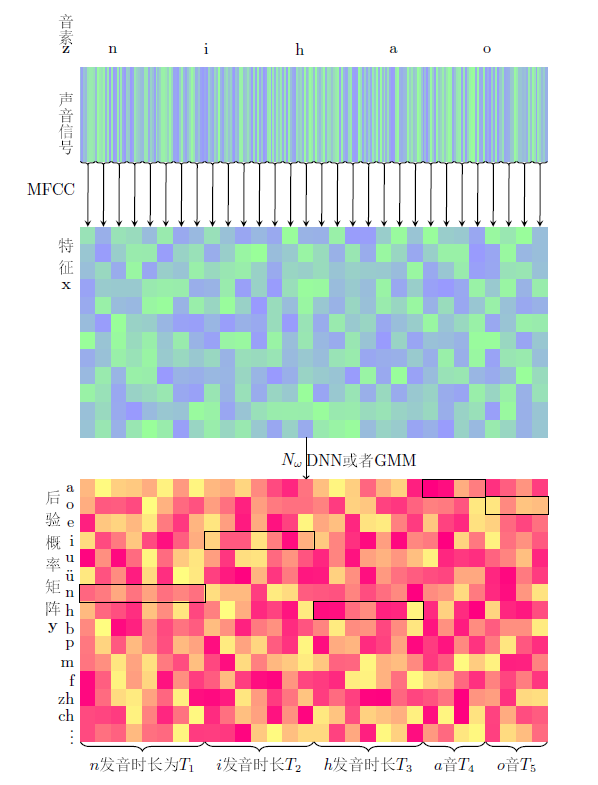以一段“你好”的语音为例，经过MFCC特征提取后产生了30帧，每帧含有12个特征，即x∈R30×14$x\in {R}^{30×14}$$x \in R^{30\times14}$(这里以14个音素为例，实际上音素有200个左右)，矩阵里的每一列之和为1。后面的基于CTC-loss的训练就是基于后验概率y$y$$y$计算得到的。

路径π$\pi$$\pi$和B变换

在实际训练中并不知道每一帧对应的音素，因此进行训练比较困难。可以先考虑一种简单的情况，已知每一帧的音素的标签z′$z\mathrm{\prime }$$z\prime$， 即训练样本为x$x$$x$和z′$z\mathrm{\prime }$$z\prime$，其中z′$z\mathrm{\prime }$$z\prime$不再是简单的[n,i,h,a,o]$\left[n,i,h,a,o\right]$$[n,i,h,a,o]$标签，而是：

z′=[n,n,n,...,nT1,i,i,i,...iT2,h,h,h,...hT3,a,a,a,...,aT4,o,o,o,...,oT5]$z\mathrm{\prime }=\left[\underset{{T}_{1}}{\underset{⏟}{n,n,n,...,n}},\underset{{T}_{2}}{\underset{⏟}{i,i,i,...i}},\underset{{T}_{3}}{\underset{⏟}{h,h,h,...h}},\underset{{T}_{4}}{\underset{⏟}{a,a,a,...,a}},\underset{{T}_{5}}{\underset{⏟}{o,o,o,...,o}}\right]$$z\prime = [\underbrace{n,n,n,...,n}_{T_1},\underbrace{i,i,i,...i}_{T_2},\underbrace{h,h,h,...h}_{T_3},\underbrace{a,a,a,...,a}_{T_4},\underbrace{o,o,o,...,o}_{T_5}]$

T1+T2+T3+T4+T5=T${T}_{1}+{T}_{2}+{T}_{3}+{T}_{4}+{T}_{5}=T$$T_1+T_2+T_3+T_4+T_5 = T$
在我们的例子中， z′=[n,n,n,n,n,n,n,i,i,i,i,i,i,h,h,h,h,h,h,h,a,a,a,a,a,a,o,o,o,o,o,o,o]$z\mathrm{\prime }=\left[n,n,n,n,n,n,n,i,i,i,i,i,i,h,h,h,h,h,h,h,a,a,a,a,a,a,o,o,o,o,o,o,o\right]$$z\prime =[n,n,n,n,n,n,n,i,i,i,i,i,i,h,h,h,h,h,h,h,a,a,a,a,a,a,o,o,o,o,o,o,o]$， z′$z\mathrm{\prime }$$z\prime$包含了每一帧的标签。在这种情况下有：
p(z′|x)=p(z′|y=Nw(x))=y1z′1y2z′2y3z′3....yTz′T$p\left(z\mathrm{\prime }|x\right)=p\left(z\mathrm{\prime }|y={N}_{w}\left(x\right)\right)={y}_{z{\mathrm{\prime }}_{1}}^{1}{y}_{z{\mathrm{\prime }}_{2}}^{2}{y}_{z{\mathrm{\prime }}_{3}}^{3}....{y}_{z{\mathrm{\prime }}_{T}}^{T}$$p(z\prime|x) = p(z\prime| y = N_w(x)) = y^1_{z\prime_1}y^2_{z\prime_2}y^3_{z\prime_3}....y^T_{z\prime_T}$      $\phantom{\rule{2em}{0ex}}\phantom{\rule{2em}{0ex}}\phantom{\rule{2em}{0ex}}\phantom{\rule{2em}{0ex}}\phantom{\rule{2em}{0ex}}\phantom{\rule{2em}{0ex}}\phantom{\rule{2em}{0ex}}\phantom{\rule{2em}{0ex}}\phantom{\rule{2em}{0ex}}\phantom{\rule{2em}{0ex}}$$\qquad\qquad\qquad\qquad\qquad\qquad\qquad\qquad\qquad\qquad$ (1)

该值即为后验概率图中用黑线圈起来的部分相乘。我们希望相乘的值越大越好，因此，数学规划可以写为：

minw−log(y1z′1.y2z′2.y3z′3...yTz′T)$mi{n}_{w}-log\left({y}_{{z}_{1}^{\mathrm{\prime }}}^{1}.{y}_{{z}_{2}^{\mathrm{\prime }}}^{2}.{y}_{{z}_{3}^{\mathrm{\prime }}}^{3}...{y}_{{z}_{T}^{\mathrm{\prime }}}^{T}\right)$$min_w -log(y^1_{z^\prime_1}.y^2_{z^\prime_2}.y^3_{z^\prime_3}...y^T_{z^\prime_T})$     $\phantom{\rule{2em}{0ex}}\phantom{\rule{2em}{0ex}}\phantom{\rule{2em}{0ex}}\phantom{\rule{2em}{0ex}}\phantom{\rule{2em}{0ex}}\phantom{\rule{2em}{0ex}}\phantom{\rule{2em}{0ex}}\phantom{\rule{2em}{0ex}}\phantom{\rule{2em}{0ex}}\phantom{\rule{2em}{0ex}}$$\qquad\qquad\qquad\qquad\qquad\qquad\qquad\qquad\qquad\qquad$ (2)

subject to: y=Nw(x)$y={N}_{w}\left(x\right)$$y = N_w(x)$$\phantom{\rule{2em}{0ex}}\phantom{\rule{2em}{0ex}}\phantom{\rule{2em}{0ex}}\phantom{\rule{2em}{0ex}}\phantom{\rule{2em}{0ex}}\phantom{\rule{2em}{0ex}}\phantom{\rule{2em}{0ex}}\phantom{\rule{2em}{0ex}}\phantom{\rule{2em}{0ex}}\phantom{\rule{2em}{0ex}}$$\qquad\qquad\qquad\qquad\qquad\qquad\qquad\qquad\qquad\qquad$ (3)

目标函数对于后验概率矩阵y$y$$y$中的每个元素ytk${y}_{k}^{t}$$y^t_k$的偏导数为：
∂−log(y1z′1.y2z′2.y3z′3...yTz′T)∂ytk=$\frac{\mathrm{\partial }-log\left({y}_{{z}_{1}^{\mathrm{\prime }}}^{1}.{y}_{{z}_{2}^{\mathrm{\prime }}}^{2}.{y}_{{z}_{3}^{\mathrm{\prime }}}^{3}...{y}_{{z}_{T}^{\mathrm{\prime }}}^{T}\right)}{\mathrm{\partial }{y}_{k}^{t}}=$$\frac{\partial - log(y^1_{z^\prime_1}.y^2_{z^\prime_2}.y^3_{z^\prime_3}...y^T_{z^\prime_T})}{\partial y^t_k} =$⎧⎩⎨⎪⎪−y1z′1...yi−1z′i−1.yi+1z′i+1....yTz′Ty1z′1.y2z′2.y3z′3...yTz′T,ifk=z′iandt=i0其他$\left\{\begin{array}{l}-\frac{{y}_{z{\mathrm{\prime }}_{1}}^{1}...{y}_{z{\mathrm{\prime }}_{i-1}}^{i-1}.{y}_{z{\mathrm{\prime }}_{i+1}}^{i+1}....{y}_{z{\mathrm{\prime }}_{T}}^{T}}{{y}_{{z}_{1}^{\mathrm{\prime }}}^{1}.{y}_{{z}_{2}^{\mathrm{\prime }}}^{2}.{y}_{{z}_{3}^{\mathrm{\prime }}}^{3}...{y}_{{z}_{T}^{\mathrm{\prime }}}^{T}},\phantom{\rule{2em}{0ex}}if\phantom{\rule{2em}{0ex}}k=z{\mathrm{\prime }}_{i}\phantom{\rule{2em}{0ex}}and\phantom{\rule{2em}{0ex}}t=i\\ 0\phantom{\rule{2em}{0ex}}其他\end{array}$$\begin{cases} -\frac{y^1_{z\prime_1}...y^{i-1}_{z\prime_{i-1}}.y^{i+1}_{z\prime_{i+1}}....y^T_{z\prime_T}}{y^1_{z^\prime_1}.y^2_{z^\prime_2}.y^3_{z^\prime_3}...y^T_{z^\prime_T}} , \qquad if \qquad k = z\prime_i \qquad and \qquad t=i \\ 0 \qquad 其他\end{cases}$

也就是说，在每个时刻t$t$$t$(对应矩阵的一列）,目标只与ytz′t${y}_{z{\mathrm{\prime }}_{t}}^{t}$$y^t_{z\prime_t}$是相关的，在这个例子中是与被框起来的元素相关。

其中Nw${N}_{w}$$N_w$可以看做是RNN模型，如果训练数据的每一帧都标记了正确的音素，那么训练过程就很简单了，但实际上这样的标记过的数据非常稀少，而没有逐帧标记的数据很多，CTC可以做到用未逐帧标记的数据做训练。

首先定义几个符号：
L={a,o,e,i,u,uˇ,b,p,m,...}$L=\left\{a,o,e,i,u,\stackrel{ˇ}{u},b,p,m,...\right\}$$L=\lbrace a, o, e, i, u, \check{u},b,p,m,...\rbrace$

表示所有音素的集合

π=(π1,π2,π3,...,πT),πi∈L$\pi =\left({\pi }_{1},{\pi }_{2},{\pi }_{3},...,{\pi }_{T}\right),{\pi }_{i}\in L$$\pi= (\pi_1, \pi_2, \pi_3, ..., \pi_T), \pi_i \in L$

表示一条由L$L$$L$中元素组成的长度为T$T$$T$的路径，比如z′$z\mathrm{\prime }$$z\prime$就是一条路径，以下为几个路径的例子：

π1=(j,j,i,n,y,y,e,e,w,w,u,u,u,r,r,e,e,n,n,r,r,u,u,sh,sh,u,u,i,i)${\pi }^{1}=\left(j,j,i,n,y,y,e,e,w,w,u,u,u,r,r,e,e,n,n,r,r,u,u,sh,sh,u,u,i,i\right)$$\pi^1= (j,j,i,n,y,y,e,e,w,w,u,u,u,r,r,e,e,n,n,r,r,u,u,sh,sh,u,u,i,i)$
π2=(n,n,n,n,i,i,i,i,h,h,h,h,a,a,a,a,a,a,a,a,a,o,o,o,o,o,o,o,o,o)${\pi }^{2}=\left(n,n,n,n,i,i,i,i,h,h,h,h,a,a,a,a,a,a,a,a,a,o,o,o,o,o,o,o,o,o\right)$$\pi^2= (n,n,n,n,i,i,i,i,h,h,h,h,a,a,a,a,a,a,a,a,a,o,o,o,o,o,o,o,o,o)$
π3=(h,h,h,h,h,h,a,a,a,a,a,a,a,o,o,o,o,n,n,n,n,n,n,i,i,i,i,i,i,i)${\pi }^{3}=\left(h,h,h,h,h,h,a,a,a,a,a,a,a,o,o,o,o,n,n,n,n,n,n,i,i,i,i,i,i,i\right)$$\pi^3= (h,h,h,h,h,h,a,a,a,a,a,a,a,o,o,o,o,n,n,n,n,n,n,i,i,i,i,i,i,i)$
π4=(n,i,h,a,o,o,o,o,o,o,o,o,o,o,o,o,o,o,o,o,o,o,o,o,o,o,o,o,o,o,o)${\pi }^{4}=\left(n,i,h,a,o,o,o,o,o,o,o,o,o,o,o,o,o,o,o,o,o,o,o,o,o,o,o,o,o,o,o\right)$$\pi^4= (n,i,h,a,o,o,o,o,o,o,o,o,o,o,o,o,o,o,o,o,o,o,o,o,o,o,o,o,o,o,o)$
π5=(n,n,n,n,n,n,n,n,n,n,n,n,n,n,n,n,n,n,n,n,n,n,n,n,i,h,a,o)${\pi }^{5}=\left(n,n,n,n,n,n,n,n,n,n,n,n,n,n,n,n,n,n,n,n,n,n,n,n,i,h,a,o\right)$$\pi^5= (n,n,n,n,n,n,n,n,n,n,n,n,n,n,n,n,n,n,n,n,n,n,n,n,i,h,a,o)$
π6=(n,n,n,i,i,i,h,h,h,h,h,a,,o,o,o,o,o,o,o,o,o,o,o,o,o,o,o,o,o,o)${\pi }^{6}=\left(n,n,n,i,i,i,h,h,h,h,h,a,,o,o,o,o,o,o,o,o,o,o,o,o,o,o,o,o,o,o\right)$$\pi^6= (n,n,n,i,i,i,h,h,h,h,h,a,,o,o,o,o,o,o,o,o,o,o,o,o,o,o,o,o,o,o)$

这6条路径中，π1${\pi }^{1}$$\pi^1$可以被认为是“今夜无人入睡”， π2${\pi }^{2}$$\pi^2$可以被认为是在说“你好”，π3${\pi }^{3}$$\pi^3$可以被认为是在说“好你”，π4,π5,π6$\pi 4,\pi 5,\pi 6$$\pi4,\pi5,\pi6$都可以认为是在说“你好”。

定义B变换，表示简单的压缩，例如：
B(a,a,a,b,b,b,c,c,d)=(a,b,c,d)$B\left(a,a,a,b,b,b,c,c,d\right)=\left(a,b,c,d\right)$$B(a,a,a,b,b,b,c,c,d) = (a,b,c,d)$

以上6条路径为例：
B(π1)=(j,i,n,y,e,w,u,r,e,n,r,u,s,h,u,i)$B\left({\pi }^{1}\right)=\left(j,i,n,y,e,w,u,r,e,n,r,u,s,h,u,i\right)$$B(\pi^1) = (j,i,n,y,e,w,u,r,e,n,r,u,s,h,u,i)$
B(π2)=(n,i,h,a,)$B\left({\pi }^{2}\right)=\left(n,i,h,a,\right)$$B(\pi^2) = (n,i,h,a,)$
B(π3)=(h,a,o,n,i)$B\left({\pi }^{3}\right)=\left(h,a,o,n,i\right)$$B(\pi^3) = (h,a,o,n,i)$
B(π4)=(n,i,h,a,o)$B\left({\pi }^{4}\right)=\left(n,i,h,a,o\right)$$B(\pi^4) = (n,i,h,a,o)$
B(π5)=(n,i,h,a,o)$B\left({\pi }^{5}\right)=\left(n,i,h,a,o\right)$$B(\pi^5) = (n,i,h,a,o)$
B(π6)=(n,i,h,a,o)$B\left({\pi }^{6}\right)=\left(n,i,h,a,o\right)$$B(\pi^6) = (n,i,h,a,o)$

因此，如果有一条路径π$\pi$$\pi$有B(π)=(n,i,h,a,o)$B\left(\pi \right)=\left(n,i,h,a,o\right)$$B(\pi)=(n,i,h,a,o)$，则可以认为π$\pi$$\pi$是在说“你好”。即使它是如π4${\pi }^{4}$$\pi^4$所示，有很多“o”的音素，而其他音素很少。路径π=(π1,π2,...,πT)$\pi =\left({\pi }^{1},{\pi }^{2},...,{\pi }^{T}\right)$$\pi = (\pi^1,\pi^2,...,\pi^T)$的概率为它所经过的矩阵y上的元素相乘：

p(π|x)=p(π|y=Nw(x))=p(π|y)=∏Tt=1ytπt$p\left(\pi |x\right)=p\left(\pi |y={N}_{w}\left(x\right)\right)=p\left(\pi |y\right)=\prod _{t=1}^{T}{y}_{{\pi }_{t}}^{t}$$p(\pi|x) = p(\pi| y = N_w(x)) = p(\pi| y)=\prod_{t=1}^{T} y^t_{\pi_t}$

因此在没有对齐的情况下，目标函数应该为{π|B(π)=z}$\left\{\pi |B\left(\pi \right)=z\right\}$$\lbrace \pi | B(\pi) = z \rbrace$中所有元素概率之和。 即：
maxwp(z|y=Nw(x))=p(z|x)=∑B(π)=zp(π|x)$ma{x}_{w}p\left(z|y={N}_{w}\left(x\right)\right)=p\left(z|x\right)=\sum _{B\left(\pi \right)=z}p\left(\pi |x\right)$$max_w p(z|y=N_w(x)) = p(z|x)= \sum_{B(\pi)=z}p(\pi|x)$     $\phantom{\rule{2em}{0ex}}\phantom{\rule{2em}{0ex}}\phantom{\rule{2em}{0ex}}$$\qquad\qquad\qquad$ (4)

在T=30，音素为[n,i,h,a,o]$\left[n,i,h,a,o\right]$$[n,i,h,a,o]$的情况下，共有C529≈120000${C}_{29}^{5}\approx 120000$$C^5_{29}\approx120000$条路径可以被压缩为[n,i,h,a,o]$\left[n,i,h,a,o\right]$$[n,i,h,a,o]$。 路径数目的计算公式为C音素个数T−1${C}_{T-1}^{音素个数}$$C^{音素个数}_{T-1}$，量级大约为(T−1)音素个数$\left(T-1{\right)}^{音素个数}$$(T-1)^{音素个数}$。一段30秒包含50个汉字的语音，其可能的路径数目可以高达108${10}^{8}$$10^8$，显然这么大的路径数目是无法直接计算的。因此CTC方法中借用了HMM中的向前向后算法来计算。

训练实施方法

CTC的训练过程是通过∂p(z|x)∂w$\frac{\mathrm{\partial }p\left(z|x\right)}{\mathrm{\partial }w}$$\frac {\partial p(z|x)}{\partial w}$调整w的值使得4中的目标值最大，而计算的过程如下：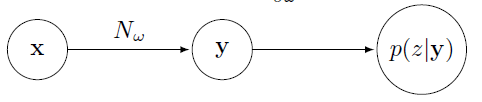因此，只要得到∂p(z|x)∂ytk$\frac{\mathrm{\partial }p\left(z|x\right)}{\mathrm{\partial }{y}_{k}^{t}}$$\frac {\partial p(z|x)}{\partial y^t_k}$，即可根据反向传播，得到∂p(z|x)∂w$\frac{\mathrm{\partial }p\left(z|x\right)}{\mathrm{\partial }w}$$\frac {\partial p(z|x)}{\partial w}$。下面以“你好”为例，介绍该值的计算方法。

首先，根据前面的例子，找到所有可能被压缩为z=[n,i,h,a,o]$z=\left[n,i,h,a,o\right]$$z=[n,i,h,a,o]$的路径，记为{π|B(π)=z}$\left\{\pi |B\left(\pi \right)=z\right\}$$\lbrace \pi|B(\pi) = z \rbrace$。 可知所有π$\pi$$\pi$均有[n,n,n,....,n,i,.....,i,h,.....h,a,....a,o,...,o]$\left[n,n,n,....,n,i,.....,i,h,.....h,a,....a,o,...,o\right]$$[n,n,n,....,n,i,.....,i,h,.....h,a,....a,o,...,o]$的形式，即目标函数只与后验概率矩阵y中表示n,i,h,a,o$n,i,h,a,o$$n,i,h,a,o$的5行相关，因此为了简便，我们将这5行提取出来，如下图所示。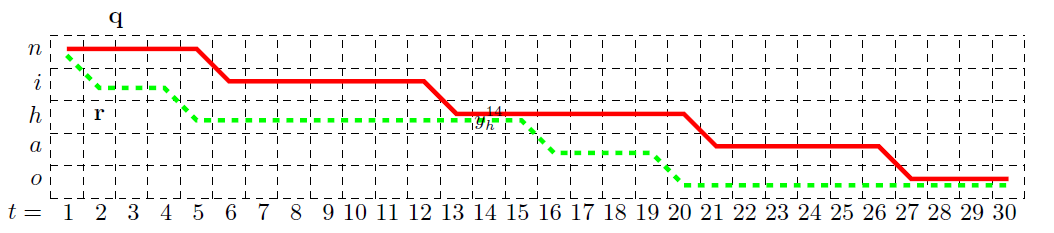在每一个点上，路径只能向右或者向下转移，画出两条路径，分别用q和r表示，这两条路径都经过y14h${y}_{h}^{14}$$y^{14}_h$这点，表示这两点路径均在第14帧的时候在发“h”音。因为在目标函数4的连加项中，有的项与y14h${y}_{h}^{14}$$y^{14}_h$无关，因此可以剔除这一部分，只留下与y14h${y}_{h}^{14}$$y^{14}_h$有关的部分，记为{π|B(π)=z,π14=h}$\left\{\pi |B\left(\pi \right)=z,{\pi }_{14}=h\right\}$$\lbrace \pi|B(\pi) = z, \pi_{14}=h \rbrace$

∂p(z|y)∂y14h$\frac{\mathrm{\partial }p\left(z|y\right)}{\mathrm{\partial }{y}_{h}^{14}}$$\frac {\partial p(z|y)}{\partial y^{14}_h}$

= ∂∑B(π)=zp(π|y)∂y14h$\frac{\mathrm{\partial }\sum _{B\left(\pi \right)=z}p\left(\pi |y\right)}{\mathrm{\partial }{y}_{h}^{14}}$$\frac {\partial \sum_{B(\pi)=z}p(\pi|y)}{\partial y^{14}_h}$

= ∂∑B(π)=z∏Tt=1ytπt∂y14h$\frac{\mathrm{\partial }\sum _{B\left(\pi \right)=z}\prod _{t=1}^{T}{y}_{{\pi }_{t}}^{t}}{\mathrm{\partial }{y}_{h}^{14}}$$\frac {\partial \sum_{B(\pi)=z}\prod_{t=1}^T y^t_{\pi_t}}{\partial y^{14}_h}$
=∂∑B(π)=z,π14=h∏Tt=1ytπt和y14h有关的项+∂∑B(π)=z,π14≠h∏Tt=1ytπt和y14h无关的项∂y14h$\frac{\stackrel{和{y}_{h}^{14}有关的项}{\stackrel{⏞}{\mathrm{\partial }\sum _{B\left(\pi \right)=z,{\pi }_{14}=h}\prod _{t=1}^{T}{y}_{{\pi }_{t}}^{t}}}+\stackrel{和{y}_{h}^{14}无关的项}{\stackrel{⏞}{\mathrm{\partial }\sum _{B\left(\pi \right)=z,{\pi }_{14}\ne h}\prod _{t=1}^{T}{y}_{{\pi }_{t}}^{t}}}}{\mathrm{\partial }{y}_{h}^{14}}$$\frac {\overbrace{\partial \sum_{B(\pi)=z,\pi_{14}=h}\prod_{t=1}^T y^t_{\pi_t}}^{和y^{14}_h有关的项} + \overbrace{\partial \sum_{B(\pi)=z,\pi_{14} \neq h}\prod_{t=1}^T y^t_{\pi_t}}^{和y^{14}_h无关的项}}{\partial y^{14}_h}$

=∂∑B(π)=z,π14=h∏Tt=1ytπt∂y14h$\frac{\mathrm{\partial }\sum _{B\left(\pi \right)=z,{\pi }_{14}=h}\prod _{t=1}^{T}{y}_{{\pi }_{t}}^{t}}{\mathrm{\partial }{y}_{h}^{14}}$$\frac {\partial \sum_{B(\pi)=z,\pi_{14}=h}\prod_{t=1}^T y^t_{\pi_t}}{\partial y^{14}_h}$

这里的q和r就是与y14h${y}_{h}^{14}$$y^{14}_h$相关的两条路径。用q1:13${q}_{1:13}$$q_{1:13}$和q15:30${q}_{15:30}$$q_{15:30}$分别表示q$q$$q$在y14h${y}_{h}^{14}$$y^{14}_h$之前和之后的部分，同样的，用r1:13${r}_{1:13}$$r_{1:13}$和r15:30${r}_{15:30}$$r_{15:30}$分别表示r$r$$r$在y14h${y}_{h}^{14}$$y^{14}_h$之前和之后的部分.。可以发现，q1:13+h+r15:30${q}_{1:13}+h+{r}_{15:30}$$q_{1:13} + h + r_{15:30}$与r1:13+h+q15:30${r}_{1:13}+h+{q}_{15:30}$$r_{1:13} + h + q_{15:30}$同样也是两条可行的路径。q1:13+h+r15:30${q}_{1:13}+h+{r}_{15:30}$$q_{1:13} + h + r_{15:30}$、r1:13+h+q15:30${r}_{1:13}+h+{q}_{15:30}$$r_{1:13} + h + q_{15:30}$、q$q$$q$ 、r$r$$r$这四条路径的概率之和为：
y1q1..y13q13.y14h.y15q15....y30q30路径q的概率$\underset{路径q的概率}{\underset{⏟}{{y}_{q1}^{1}..{y}_{q13}^{13}.{y}_{h}^{14}.{y}_{q15}^{15}....{y}_{q30}^{30}}}$$\underbrace{y^1_{q1}..y^{13}_{q13}.y^{14}_{h}.y^{15}_{q15}....y^{30}_{q30}}_{路径q的概率}$

+y1q1..y13q13.y14h.y15r15....y30r30路径q1:14+r14:30的概率$\underset{路径{q}_{1:14}+{r}_{14:30}的概率}{\underset{⏟}{{y}_{q1}^{1}..{y}_{q13}^{13}.{y}_{h}^{14}.{y}_{r15}^{15}....{y}_{r30}^{30}}}$$\underbrace{y^1_{q1}..y^{13}_{q13}.y^{14}_{h}.y^{15}_{r15}....y^{30}_{r30}}_{路径q_{1:14}+r_{14:30}的概率}$

+y1r1..y13r13.y14h.y15q15....y30q30路径r1:14+q14:30的概率$\underset{路径{r}_{1:14}+{q}_{14:30}的概率}{\underset{⏟}{{y}_{r1}^{1}..{y}_{r13}^{13}.{y}_{h}^{14}.{y}_{q15}^{15}....{y}_{q30}^{30}}}$$\underbrace{y^1_{r1}..y^{13}_{r13}.y^{14}_{h}.y^{15}_{q15}....y^{30}_{q30}}_{路径r_{1:14}+q_{14:30}的概率}$

+y1r1..y13r13.y14h.y15r15....y30r30路径r的概率$\underset{路径r的概率}{\underset{⏟}{{y}_{r1}^{1}..{y}_{r13}^{13}.{y}_{h}^{14}.{y}_{r15}^{15}....{y}_{r30}^{30}}}$$\underbrace{y^1_{r1}..y^{13}_{r13}.y^{14}_{h}.y^{15}_{r15}....y^{30}_{r30}}_{路径r的概率}$

=(y1q1....y13q13+y1r1.....y13r13).y14h.(y15q15....y30q15+y15r15....y30r30)$\left({y}_{q1}^{1}....{y}_{q13}^{13}+{y}_{r1}^{1}.....{y}_{r13}^{13}\right).{y}_{h}^{14}.\left({y}_{q15}^{15}....{y}_{q15}^{30}+{y}_{r15}^{15}....{y}_{r30}^{30}\right)$$(y^1_{q1}....y^{13}_{q13} + y^1_{r1}.....y^{13}_{r13}).y^{14}_h.(y^{15}_{q15}....y^{30}_{q15}+y^{15}_{r15}....y^{30}_{r30})$

可以发现，该值可以总结为：（前置项）.y14h.$.{y}_{h}^{14}.$$.y^{14}_h.$(后置项)。由此，对于所有的经过y14h${y}_{h}^{14}$$y^{14}_h$的路径，有：

∑B(π)=z,π14=h∏Tt=1ytπt=(前置项).y14h.$\sum _{B\left(\pi \right)=z,{\pi }_{14}=h}\prod _{t=1}^{T}{y}_{{\pi }_{t}}^{t}=\left(前置项\right).{y}_{h}^{14}.$$\sum_{B(\pi)=z,\pi_{14}=h}\prod_{t=1}^T y^t_{\pi_t}= (前置项).y^{14}_h.$(后置项)$定义： α(14)(h)=(前置项).y14h=∑B(π1:14)=[n,i,h]∏tt′=1yt′πt′${\alpha }_{\left(}14\right)\left(h\right)=\left(前置项\right).{y}_{h}^{14}=\sum _{B\left({\pi }_{1:14}\right)=\left[n,i,h\right]}\prod _{t\mathrm{\prime }=1}^{t}{y}_{{\pi }_{t\mathrm{\prime }}}^{t\mathrm{\prime }}$$\alpha_(14)(h)=(前置项).y^{14}_h = \sum_{B(\pi_{1:14})=[n,i,h] }\prod_{t\prime=1}^t y^{t\prime}_{\pi_{t\prime}}$ 该值可以理解为从初始到y14h${y}_{h}^{14}$$y^{14}_h$这一段里，所有正向路径的概率之和。并且发现，α14(h)${\alpha }_{14}\left(h\right)$$\alpha_{14}(h)$可以由α13(h)${\alpha }_{13}\left(h\right)$$\alpha_{13}(h)$和α13(i)${\alpha }_{13}\left(i\right)$$\alpha_{13}(i)$递推得到，即： α14(h)=(α13(h)+α13(i))y14h${\alpha }_{14}\left(h\right)=\left({\alpha }_{13}\left(h\right)+{\alpha }_{13}\left(i\right)\right){y}_{h}^{14}$$\alpha_{14}(h) = (\alpha_{13}(h) + \alpha_{13}(i))y^{14}_h$ 该递推公式的含义是，只是在t=13$t=13$$t=13$时发音是“h”或“i”，在t=14$t=14$$t=14$时才有可能发音是“h”。那么在t=14$t=14$$t=14$时刻发音是“h”的所有正向路径概率α14(h)${\alpha }_{14}\left(h\right)$$\alpha_{14}(h)$就等于在t=13$t=13$$t=13$时刻，发音为“h”的正向概率α13(h)${\alpha }_{13}\left(h\right)$$\alpha_{13}(h)$加上发音为“i”的正向概率α13(i)${\alpha }_{13}\left(i\right)$$\alpha_{13}(i)$，再乘以当前音素被判断为“h”的概率y14h${y}_{h}^{14}$$y^{14}_h$。由此可知，每个αt(s)${\alpha }_{t}\left(s\right)$$\alpha_t(s)$都可以由αt−1(s)${\alpha }_{t-1}\left(s\right)$$\alpha_{t-1}(s)$和αt−1(s−1)${\alpha }_{t-1}\left(s-1\right)$$\alpha_{t-1}(s-1)$两个值得到。α$\alpha$$\alpha$的递推流程如下图所示：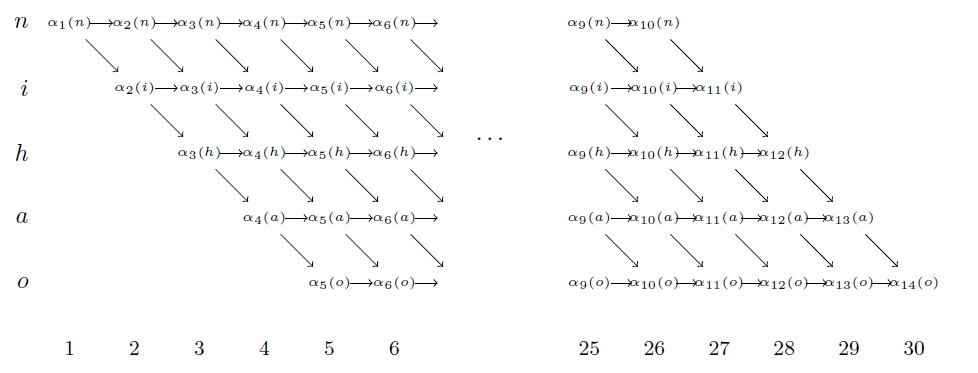即每个值都由上一个时刻的一个或者两个值得到，总计算量大约为2.T.音素个数$2.T.音素个数$$2.T.音素个数$。类似的，定义βt(s)${\beta }_{t}\left(s\right)$$\beta_t(s)$， 递推公式为： β14(h)=(β15(h)+β15(a))y14h${\beta }_{14}\left(h\right)=\left({\beta }_{15}\left(h\right)+{\beta }_{15}\left(a\right)\right){y}_{h}^{14}$$\beta_{14}(h)=(\beta_{15}(h) + \beta_{15}(a))y^{14}_h$ 因此有： ∑B(π)=z,π14=h∏Tt=1ytπt=(前置项).y14h.$\sum _{B\left(\pi \right)=z,{\pi }_{14}=h}\prod _{t=1}^{T}{y}_{{\pi }_{t}}^{t}=\left(前置项\right).{y}_{h}^{14}.$$\sum_{B(\pi)=z,\pi_{14}=h}\prod_{t=1}^T y^t_{\pi_t}= (前置项).y^{14}_h.$(后置项)$

=α14(h)y14h.y14h.β14(h)y14h$\frac{{\alpha }_{14}\left(h\right)}{{y}_{h}^{14}}.{y}_{h}^{14}.\frac{{\beta }_{14}\left(h\right)}{{y}_{h}^{14}}$$\frac{\alpha_{14}(h)}{y^{14}_h}. y^{14}_h.\frac{\beta_{14}(h)}{y^{14}_h}$

=α14(h)β14(h)y14h$\frac{{\alpha }_{14}\left(h\right){\beta }_{14}\left(h\right)}{{y}_{h}^{14}}$$\frac{\alpha_{14}(h)\beta_{14}(h)}{y^{14}_h}$

然后：

∂p(z|y)∂y14h$\frac{\mathrm{\partial }p\left(z|y\right)}{\mathrm{\partial }{y}_{h}^{14}}$$\frac {\partial p(z|y)}{\partial y^{14}_h}$

= ∑B(π)=z,π14=h∏Tt=1ytπt∂y14h$\frac{\sum _{B\left(\pi \right)=z,{\pi }_{14}=h}\prod _{t=1}^{T}{y}_{{\pi }_{t}}^{t}}{\mathrm{\partial }{y}_{h}^{14}}$$\frac {\sum_{B(\pi)=z,\pi_{14}=h}\prod_{t=1}^T y^t_{\pi_t}}{\partial y^{14}_h}$

=  α14(h)y14h.y14h.β14(h)y14h∂y14h$\frac{\frac{{\alpha }_{14}\left(h\right)}{{y}_{h}^{14}}.{y}_{h}^{14}.\frac{{\beta }_{14}\left(h\right)}{{y}_{h}^{14}}}{\mathrm{\partial }{y}_{h}^{14}}$$\frac {\frac{\alpha_{14}(h)}{y^{14}_h}. y^{14}_h.\frac{\beta_{14}(h)}{y^{14}_h}}{\partial y^{14}_h}$

=α14(h)β14(h)(y14h)2$\frac{{\alpha }_{14}\left(h\right){\beta }_{14}\left(h\right)}{{\left({y}_{h}^{14}\right)}^{2}}$$\frac{\alpha_{14}(h)\beta_{14}(h)}{{(y^{14}_h)}^2}$

得到此值后，就可以根据反向传播算法进行训练了。
可以看到，这里总的计算量非常小，计算α$\alpha$$\alpha$和β$\beta$$\beta$的计算量均大约为(2.T.音素个数)$\left(2.T.音素个数\right)$$(2.T.音素个数)$，（加法乘法各一次），得到α$\alpha$$\alpha$和β$\beta$$\beta$之后，在计算对每个ytk${y}_{k}^{t}$$y^t_k$的偏导值的计算量为(3.T.音素个数)$\left(3.T.音素个数\right)$$(3.T.音素个数)$，因此总计算量大约为(7.T.音素个数)$\left(7.T.音素个数\right)$$(7.T.音素个数)$，这是非常小的，便于计算。

目前，深度学习的算法已经大规模应用于腾讯云的语音识别产品中。腾讯云拥有业内最先进的语音识别技术，基于海量的语音数据，积累了数十万小时的标注语音数据，采用LSTM，CNN，LFMMI，CTC等多种建模技术，结合超大规模语料的语言模型，对标准普通话的识别效果超过了97%的准确率。腾讯云的语音技术，应用涵盖范围广泛，具备出色的语音识别、语音合成、关键词检索、静音检测、语速检测、情绪识别等能力。并且针对游戏，娱乐，政务等几十个垂直做特殊定制的语音识别方案，让语音识别的效果更精准，更高效，全面满足电话客服质检、语音听写、实时语音识别和直播字幕等多种场景的应用。
展开全文深度学习 语音识别
• ## CTC算法详解

万次阅读 多人点赞 2018-11-02 19:49:59
和其它文章初衷一样，网上解释很多，还有原文，但是讲的不是很明白，在看完几篇参考博客后特此记录 简介 先拿语音识别任务来说，如果现在有一个包含剪辑语音和对应的文本，我们不知道如何将语音片段与文本进行对应...
和其它文章初衷一样，网上解释很多，但是讲的不是很明白，在看完几篇参考博客后特此记录
简介
先拿语音识别任务来说，如果现在有一个包含剪辑语音和对应的文本，我们不知道如何将语音片段与文本进行对应，这样对于训练一个语音识别器增加了难度。
为了解决上述问题，我们可以先制定一个规则，例如“一个字符对于是个语言片段输入”。对于不同的人来说，他们说话的语速也不一样，这样导致了上述的定义规则不可行。另一个解决办法，手动对齐每个字符在音频中的位置。这种方法对于我们训练模型非常有效，但是不可否认的是这种做法非常耗时。
上面只是拿语音识别来举例，其实在其他一些识别任务中也会出现这个问题，例如手写字符识别，上面两例如下图所示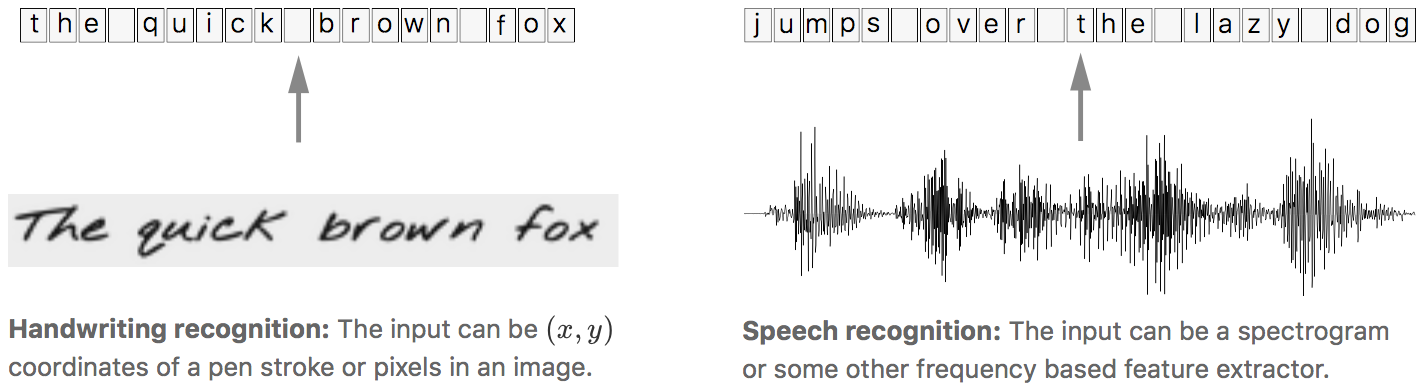Connectionist Temporal Classification (CTC)正适合这种不知道输入输出是否对齐的情况使用的算法，所以CTC适合语音识别和手写字符识别的任务
为了方便下面的描述，我们做如下定义，输入(如音频信号)用符号序列$X=[x_{1},x_{2},...,x_{T}]$表示，对应的输出(如对应的标注文本)用符号序列$Y=[y_{1},y_{2},...,y_{U}]$，为了方便训练这些数据我们希望能够找到输入$X$与输出$Y$之间精确的映射关系。
在使用有监督学习算法训练模型之前，有几个难点：

$X$和$Y$都是变长的
$X$和$Y$的长度比也是变化的
$X$和$Y$相应的元素之间没有严格的对齐(即$x_{t}$与$y_{u}$不一定对齐)

使用CTC算法能克服上述问题。到这里可以知道CTC就是可以解决输入输出对应问题的一种算法。
这里我们首先需要明确的是，还拿语音识别来说，现在使用的CTC常用的场景是RNN后接CTC算法，RNN模型输入是个个音频片段，输出个数与输入的维度一样，有T个音频片段，就输出T个维度的概率向量，每个向量又由字典个数的概率组成。例如网络输入音频个数定为T，字典中不同字的个数为N，那么RNN输出的维度为$T\times N$。根据这个概率输出分布，我们就能得到最可能的输出结果。在接下来的讨论中可以把RNN+CTC看成一个整体，当然也可以将RNN替换成其他的提取特征算法
损失函数的定义： 对于给定的输入$X$，我们训练模型希望最大化$Y$的后验概率$P(Y|X)$,$P(Y|X)$应该是可导的，这样我们就能利用梯度下降训练模型了。
测试阶段： 当我们已经训练好一个模型后，输入$X$，我们希望输出$Y$的条件概率最高即$Y^{*}=\mathop{\arg\max}_{Y}p(Y|X)$，而且我们希望尽量快速的得到$Y^{*}$值，利用CTC我们能在低投入情况下迅速找到一个近似的输出。
算法
CTC算法对于输入的$X$能给出非常多的Y的条件概率输出(可以想象RNN输出概率分布矩阵，所以通过矩阵中元素的组合可以得到很多Y值作为最终输出)，在计算输出过程的一个关键问题就是CTC算法如何将输入和输出进行对齐的。在接下来的部分中，我们先来看一下对齐的解决方法，然后介绍损失函数的计算方法和在测试阶段中找到合理输出的方法。
对齐
CTC算法并不要求输入输出是严格对齐的。但是为了方便训练模型我们需要一个将输入输出对齐的映射关系，知道对齐方式才能更好的理解之后损失函数的计算方法和测试使用的计算方法。
为了更好的理解CTC的对齐方法，先举个简单的对齐方法。假设对于一段音频，我们希望的输出是$Y=[c,a,t]$这个序列，一种将输入输出进行对齐的方式如下图所示，先将每个输入对应一个输出字符，然后将重复的字符删除。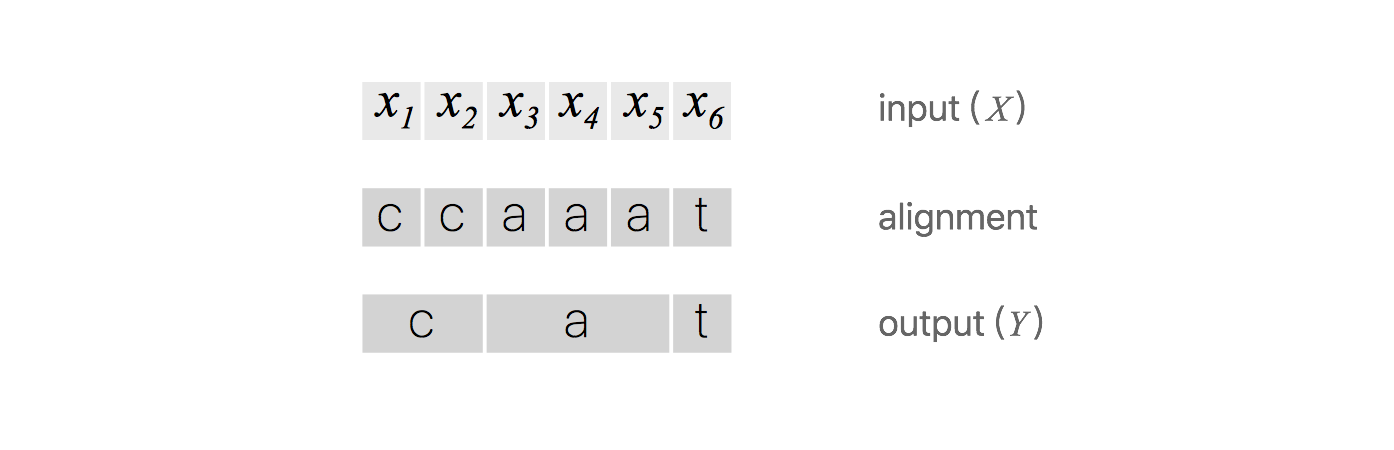上述对齐方式有两个问题：

通常这种对齐方式是不合理的。比如在语音识别任务中，有些音频片可能是无声的，这时候应该是没有字符输出的
对于一些本应含有重复字符的输出，这种对齐方式没法得到准确的输出。例如输出对齐的结果为$[h,h,e,l,l,l,o]$，通过去重操作后得到的不是“hello”而是“helo”

为了解决上述问题，CTC算法引入的一个新的占位符用于输出对齐的结果。这个占位符称为空白占位符，通常使用符号$\epsilon$，这个符号在对齐结果中输出，但是在最后的去重操作会将所有的$\epsilon$删除得到最终的输出。利用这个占位符，可以将输入与输出有了非常合理的对应关系，如下图所示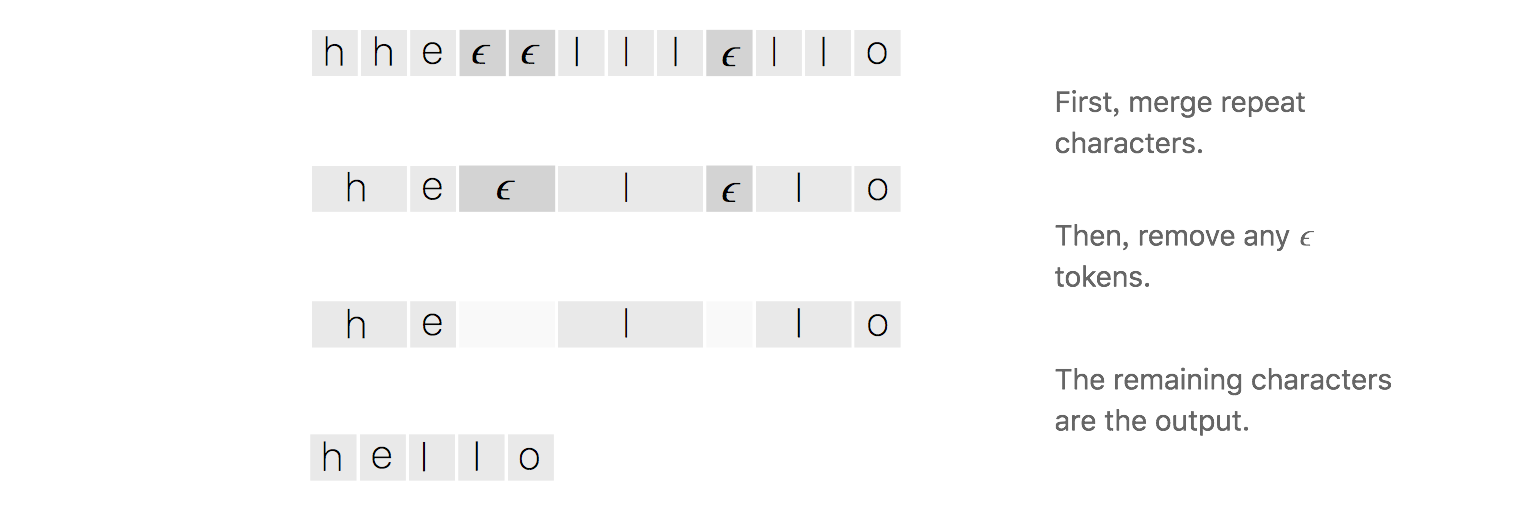在这个映射方式中，如果在标定文本中有重复的字符，对齐过程中会在两个重复的字符当中插入$\epsilon$占位符。利用这个规则，上面的“hello”就不会变成“helo”了。
回到上面$Y=[c,a,t]$这个例子来，下图中有几个示列说明有效的对齐方式和无效的对齐方式，在无效的对齐方式中举了三种例子，占位符插入位置不对导致的输出不对，输出长度与输入不对齐，输出缺少字符a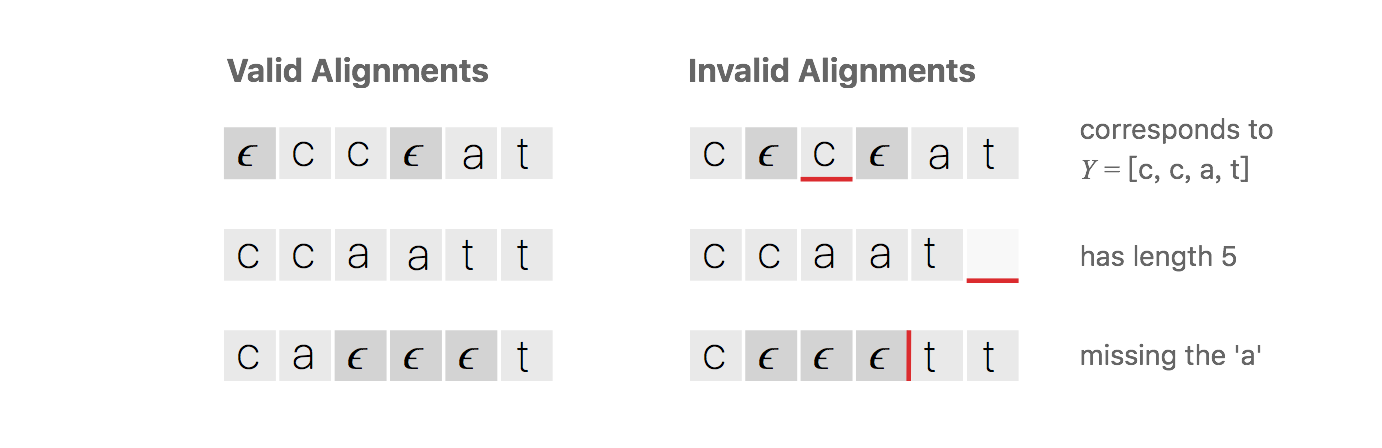CTC算法的对齐方式有下列属性：

输入与输出的对齐方式是单调的，即如果输入下一输入片段时输出会保持不变或者也会移动到下一个时间片段
输入与输出是多对一的关系
输出的长度小于等于输入

损失函数
这里要明确一点，对于一个标定好的音频片段，训练该片段时，我们希望的输出就是标定的文本，如下图所示，音频说的一个hello，RNN或者其他模型输出的是相同数量的向量，向量里是每个字母的概率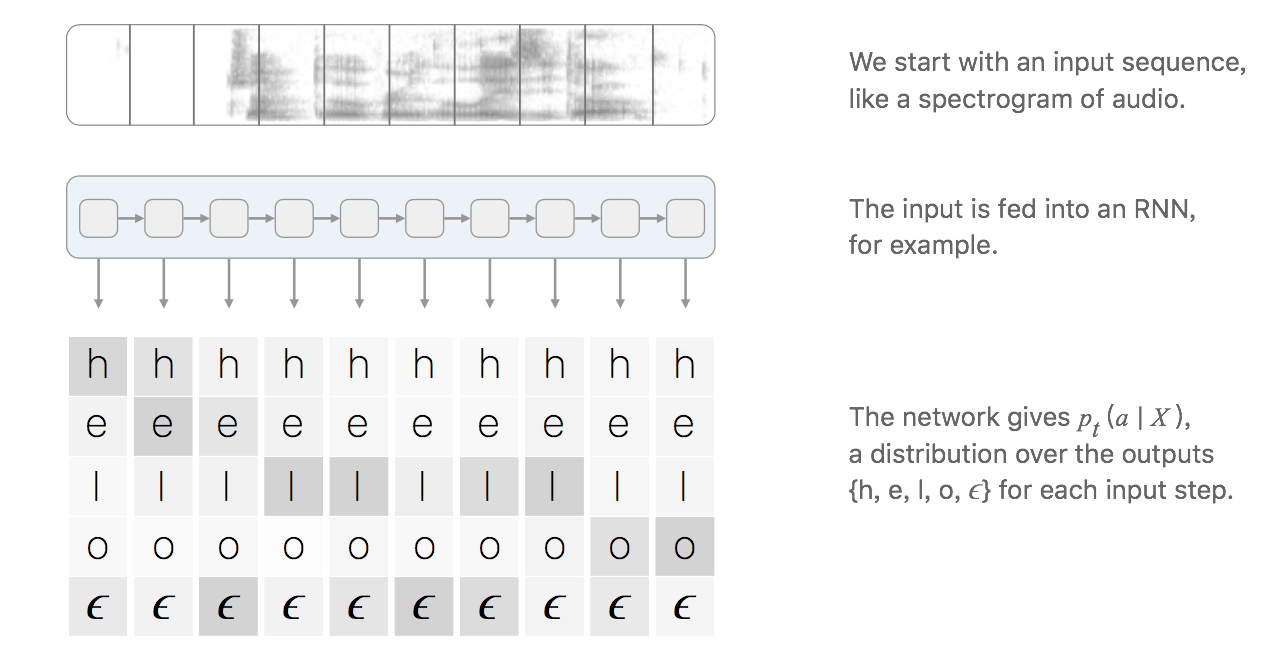对于一对输入输出$(X,Y)$来说，CTC的目标是将下式概率最大化
$p(Y|X)=\sum_{A\in\mathcal{A}_{X,Y}} \prod^{T}_{t=1}p_{t}(a_{t}|X)$
解释一下，对于RNN+CTC模型来说，RNN输出的就是$p_{t}(a_{t}|X)$概率，t表示的是RNN里面的时间的概念。乘法表示一条路径的所有字符概率相乘，加法表示多条路径。因为上面说过CTC对齐输入输出是多对一的，例如$he\epsilon l\epsilon lo\epsilon$与$hee\epsilon l\epsilon lo$对应的都是“hello”，这就是输出的其中两条路径，要将所有的路径相加才是输出的条件概率
但是对于一个输出，路径会非常的多，这样直接计算概率是不现实的，CTC算法采用动态规划的思想来求解输出的条件概率，如下图所示，该图想说明的是通过动态规划来进行路径的合并(看不懂也没关系，下面有详细的解释)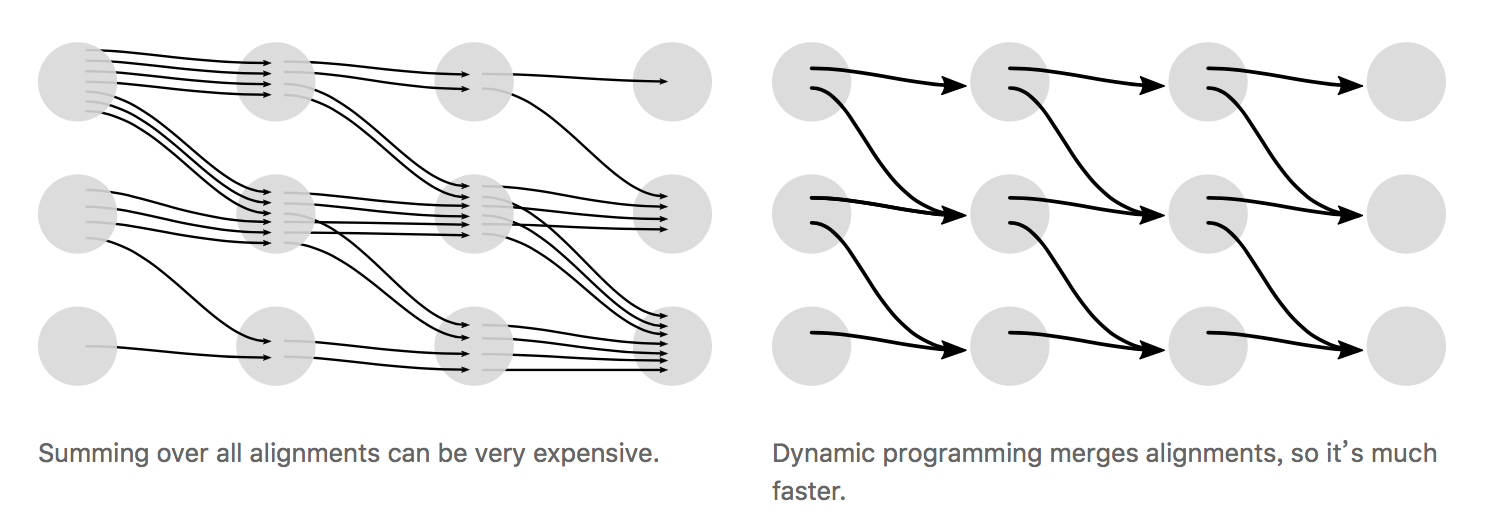假设我们现在有输入音频$X$对应的标定输出$Y$为单词“ZOO”，为了方便解释下面动态规划的思想，现在每个字符之间还有字符串的首位插入空白占位符$\epsilon$，得到下面结果
$Z=\{\epsilon,Z,\epsilon,O,\epsilon,O,\epsilon\}$
为了便于说明，先定义好下图的横纵坐标轴的含义，横轴是$X$的时间片单位为t，纵轴为$Z$序列单位为s。根据CTC的对齐方式的三个特征，输入有9个时间片，标签内容是“ZOO”，$P(Y|X)$的所有可能的合法路径如下图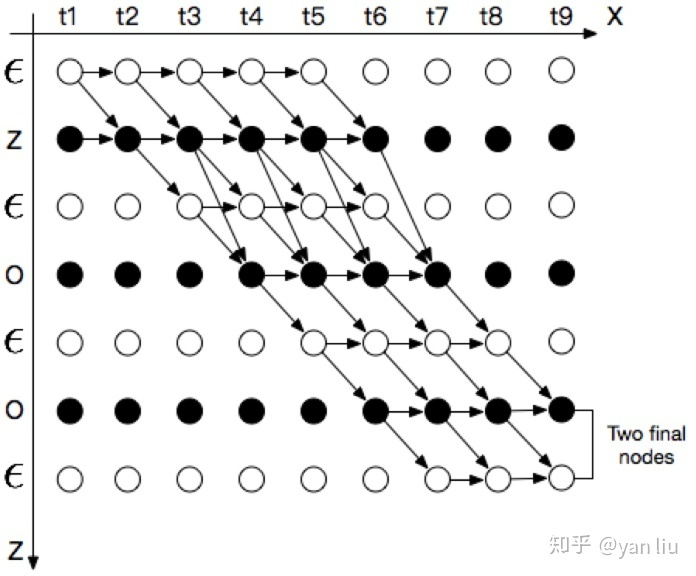$\alpha$表示对齐结果合并后(如图3.png)节点的概率。$\alpha_{s,t}$表示上图中坐标为(s,t)节点的概率，该点的概率计算分为下面两种情况：
Case 1：
1）如果$\alpha_{s,t}=\epsilon$，则$\alpha_{s,t}$只能由前一个字符$\alpha_{s-1,t-1}$或者本身$\alpha_{s,t-1}$得到
2）如果$\alpha_{s,t}$不等于$\epsilon$，但是$\alpha_{s,t}$为连续字符的第二个，即$\alpha_{s}=\alpha_{s-2}$($\alpha_{s-1}=\epsilon$)，则$\alpha_{s,t}$只能由一个空白符$\alpha_{s-1,t-1}$或者其本身$\alpha_{s,t-1}$得到，而不能由前一个字符得到。
上述两种情况中，$\alpha_{s,t}$可以由下式算出，其中$p_{t}(z_{s}|X)$表示在时刻t输出字符$z_{s}$的概率。
$\alpha_{s,t}=(\alpha(s,t-1)+\alpha(s-1,t-1))\cdot p_{t}(z_{s}|X)$
Case 2：
如果$\alpha_{s,t}$不等于$\epsilon$，则$\alpha_{s,t}$可以由$\alpha_{s,t-1}$，$\alpha_{s-1,t-1}$以及$\alpha_{s-2,t-1}$得来，可以表示为
$\alpha_{s,t}=(\alpha(s,t-1)+\alpha(s-1,t-1)+\alpha(s-2,t-1))\cdot p_{t}(z_{s}|X)$
从图7中可以看到合法路径由两个起始点，输出两个终止点，最后输出的条件概率为两个终止点输出概率的和。使用这种计算方法就能高效的计算损失函数，下一步的工作表示计算梯度用于训练模型。由于P(Y|X)的计算只涉及加法和乘法，因此是可导的。对于训练集$\mathcal{D}$，模型优化的目标是最小化负对数似然函数
$\sum_{(X,Y)\in \mathcal{D} }-logp(Y|X)$
预测
当我们训练好一个模型后，我们输入$X$，我们的目的是计算下式得到输出
$Y*=\mathop{\arg\max}_{Y}p(Y|X)$
1.一种方法是贪婪算法，取RNN每次输出概率最大的节点，计算方式如下
$A*=\mathop{\arg\max}_{A} \prod^{T}_{t=1}p_{t}(a_{t}|X)$
然后通过去重得到输出结果。
通常这种启发式的算法很有效，但是这种方法忽略了一个输出可能对应多个对齐结果。例如$[a,a,\epsilon]$和$[a,a,a]$各自的概率均小于$[b,b,b]$的概率，但是他们相加的概率比$[b,b,b]$概率高。简单的启发是算法得到结果为$Y=[b]$，但是结果为$Y=[a]$更为合理。考虑到这点第二种方式变的更为合理
2.第二种算法是Beam search的一种变形
先来说一下Beam search算法，该算法有个参数叫做宽度，假设宽度设为3，在RNN的输出中，该算法每个时间t输出时，不同于贪婪算法只找最高的，而是找最高的三个概率作为下一次的输入，依次迭代，如下图所示，每次t时间都是基于t-1输出的最高三个查找当前概率最高的三个。(这里也可以看出，当宽度设置为1时就是贪婪算法)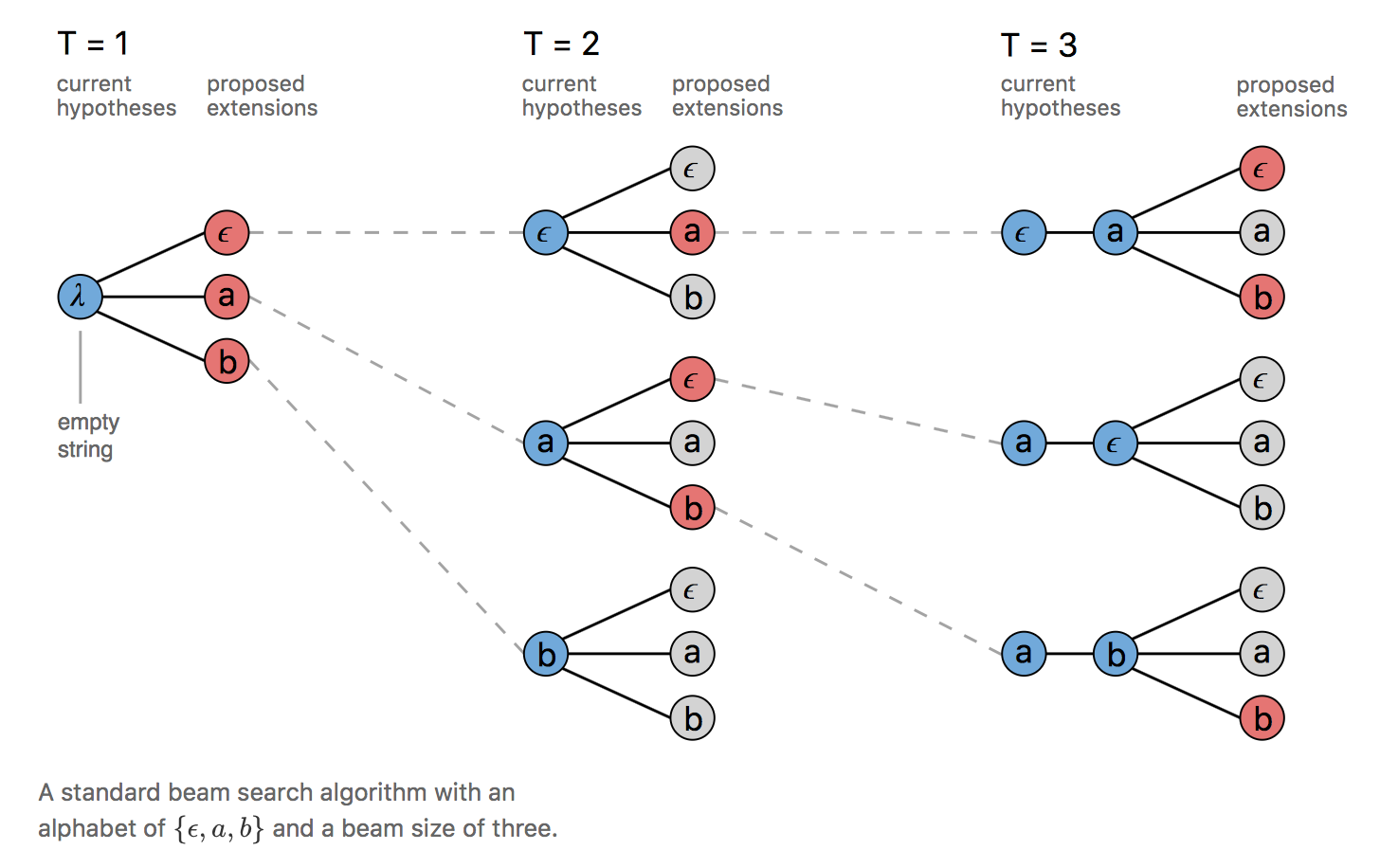因为我们这里想要结合多个对齐能够映射到同一输出的这种情况，这时每次t时间的输出为去重后以及移除$\epsilon$的结果，具体如下图所示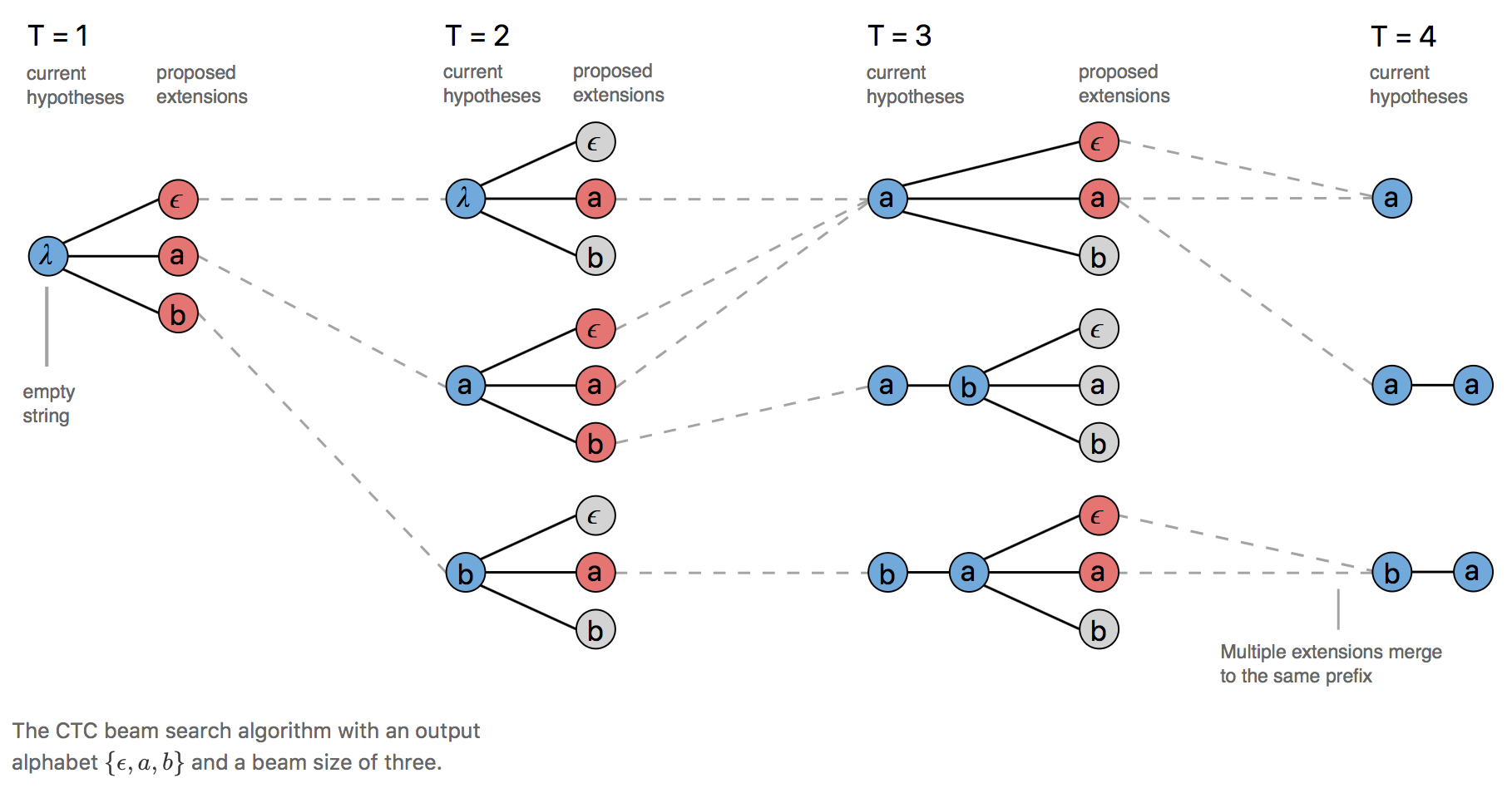当输出的前缀字符串遇上重复字符时，可以映射到两个输出，如图9所示，当T=3时，前缀包含a，遇上新的a，则[a]和[a,a]两个输出都是有效的。
当我们将[a]扩展为[a, a]时，我们只需统计之前以空白标记$\epsilon$结尾的所有路径的概率（位于字符中间的ϵ也要统计）。同样的，如果是扩展到[a]，那我们计算的就是不以$\epsilon$结尾的所有路径概率。所以每次的输出只需要记录空白标记$\epsilon$结尾的所有路径的概率和不以$\epsilon$结尾的所有路径概率来进行下一次的概率计算。这个算法的实现，Awni Hannun给出了示例
CTC的特征

条件独立：CTC的一个非常不合理的假设是其假设每个时间片都是相互独立的，这是一个非常不好的假设。在OCR或者语音识别中，各个时间片之间是含有一些语义信息的，所以如果能够在CTC中加入语言模型的话效果应该会有提升。
单调对齐：CTC的另外一个约束是输入$X$与输出$Y$之间的单调对齐，在OCR和语音识别中，这种约束是成立的。但是在一些场景中例如机器翻译，这个约束便无效了。
多对一映射：CTC的又一个约束是输入序列$X$的长度大于标签数据 $Y$的长度，但是对于$X$的长度大于$Y$的长度的场景，CTC便失效了。

参考
 https://distill.pub/2017/ctc/
 https://gist.github.com/awni/56369a90d03953e370f3964c826ed4b0
 https://zhuanlan.zhihu.com/p/42719047
 https://www.zhihu.com/question/47642307
 https://www.cs.toronto.edu/~graves/icml_2006.pdf
欢迎加入OCR交流群：785515057（此群已满）
欢迎加入OCR交流群2：826714963


展开全文机器学习
• ## CTC 介绍

千次阅读 2017-11-21 21:25:26
[论文]CTC——Connectionist Temporal Classification: Labelling Unsegmented Sequence Data with Recurrent Neural Networks   EdwardLee 关注 2016.08.31 12:46 字数 3257 阅读 4793评论 1喜欢 7 写在...

[论文]CTC——Connectionist Temporal Classification: Labelling Unsegmented Sequence Data with Recurrent Neural Networks

http://blog.csdn.net/App_12062011/article/details/76886954

PIL时的依赖比较多，在build前最好build_ext -i一下，看下需要的依赖是不是都安装好了。
论文地址
icml2006
摘要：
许多真实世界中的序列学习任务，往往需要从噪声和未格式化的数据上，预测序列的label。例如，在语音识别中，一个声音信号被转换成words或者是sub-word单元。RNN是序列学习的一个强大的模型。但是，它要求预先分割（pre-segmented）的训练数据，通过后处理将模型输出转换为label序列，因此它的应用受到较大的限制。这篇文章提出了一个新的RNN训练方法，支持直接对未分割的序列上预测label。在TIMIT语料上，对比了HMM和HMM-RNN构成Baseline的效果。
一、介绍
label未分割的序列数据是一个普遍存在序列学习任务。尤其是在感知任务中，比如手写识别、语音识别、手势识别等，这些场景中噪声、真实输入数据流将被离散字符label表达，例如letters或者words。
目前，图模型例如HMM、CRF以及它们的变体，在序列label领域都是很有影响力的框架。虽然在很多问题上，这些方法都得到了很好的证明，但是他们仍然存在缺陷：
1）他们往往需要大量的任务相关的知识，例如涉及HMM的状态模型，或者选择CRF的输入特征。
2）他们往往依赖显示的模型假设，来保证推理inference容易处理，例如HMM的观察独立性假设。
3）对于标准的HMM，训练是生成式的，但是序列标注任务是判别式的。
在另外一方面，RNN模型在输入和输出的表达选择外，对数据不需要任何的先验知识prior knowledge。并且，通过判别式的方式训练，内部internal状态提供了强大且通用的机制来建模时间序列。此外，它对时间temporal和空间spatial上的噪声表现出鲁棒性。
但是，到目前为止，我们还不能将RNN直接应用到序列标注任务上。问题在于，标准的neural network的目标函数，是在训练序列的每个节点上，单独定义的。换句话说，RNN只能被用来处理一系列独立label分类任务。这意味着，训练数据必须是预先分割的，网络输出必须经过后处理来给出最终的label序列。
目前，序列label任务里最有效的使用RNN的方法，是与HMM结合在一起，构成所谓的Hybrid方法（Bourlard&Morgan；Bengio）。Hybrid系统使用HMM来建模数据中的long-range序列结构，使用neural nets来提供局部分类能力。HMM部分可以在训练过程中自动的分割segment序列，将网络分类转换成标签序列label
sequence。但是，同样继承了HMM的缺点，hybrid系统没有充分利用RNN在序列模型上的潜力。
这篇文章提出了使用RNN标注序列数据的新方法，避免了对训练数据预先分隔以及输出后处理的要求，仅采用一个单独的网络架构建模对序列的全部方面进行建模。最基本的思路是将网络输出解释为，在给定输入下，所有可能对应的label序列上的一个概率分布。给定这个分布，目标函数可以是直接最大化正确label的概率。因为目标函数是可导的，网络可以通过标准的BP方法来训练（Werbos，1990）。
classification。
下一个章节给出了temporal分类的数据公式，定义了本文使用的误差度量方法。第3节描述了输出表示，允许使用RNN完成temporal 分类。第4节中解释了CTC网络如何训练。第5节比较了CTC和hybrid以及HMM系统。第6节讨论了CTC与其他temporal分类的一些关键区别，以及未来工作的规划。第7节是论文结尾。
二、时序分类Temporal classification
S代表训练集合，符合分布Dx×z。输入空间X=(Rm)*，代表m维具有真实值的向量，构成的序列的集合。目标空间Z=L*，代表标签Label的有限字符集合构成的序列的集合。一般来说，我们把L*中的元素看做是label序列或标签。S中的每个样本构成一个序列对（x,z）。目标序列Z=(z1,..zu)的长度至多与输入序列X=(x1,…,xt)等长，即U≤T。由于输入和目标序列一般都是不等长的，因此没有先验的方法可以对齐。
我们的目的是利用S来训练一个时序分类h:X->X，对未知的输入序列分类，最小化一些任务对应的误差量化指标。
1、标签错误率Label Error Rate
在这篇论文中，错误率的度量是比较关键的。考虑一个测试集合S∈Dx×z，定义时序分类h的标签错误率LER（Label error rate）为：分类结果和目标的平均归一化编辑距离ED(p,q)表示p和q两个序列的编辑距离。例如，将p变为q需要的最小的插入、替换和删除数。
对于目标是减少转换错误率的任务（比如语音和手写识别）来说是最自然的度量方法。
三、CTC——Connectionist Temporal Classification
本节将描述使RNN支持CTC模型所需要的输出表示。关键步骤是将网络输出转换为一个在label序列上的条件概率分布。之后对于给定输入，网络通过选择最可能的label来完成分类。
1、从网络输出到Labellings
一个CTC网络具有softmax 输出层，该层比label集合L多出一个unit。对于|L|个units的触发被解释为在特定的时刻观察到对应的label的概率，对于多余的unit的出发被看做观察到空格或者no label的概率。总的来说，这些输出定义了将label序列对齐到输入序列的全部可能方法的概率。任何一个label序列的总概率，可以看做是它的不同对齐形式对应的全部概率累加。
更加正式的，对于一个给定的输入序列X，长度为T，定义一个RNN网络，m个输入，n个输出，权重向量w是一个连续映射Nw：(R^m)^T -> (R^n)^T。设Y=Nw(x)为网络的输出序列，y(k,t)表示输出单元k在t时刻被触发，被解释为在t时刻观察到label k的概率，这个观察定义了在字符集合L=L∪{blank}的长度为T的序列的集合L^T的概率分布。现在，我们把L^T中的元素看做路径paths并且用π表示。公式（2）的假设是，给定网络的中间状态（internal state），在不同时刻的网络输出是条件独立的。这保证了输出层不存在到它自身或者网络的反馈链接。
下一步是定义一个多对一的映射β：L^T -> L^≤T，其中后者是可能的label序列的集合。我们可以简单通过删除全部的blank和重复路径path中的label来实现，例如β(a-ab-)=β(-aa—abb)=aab
直觉地，这等价于输出一个新的label，从预测no label变为预测a label，或者从预测a label到预测另外一个label。参考Figure1最终，我们用映射β来定义给定一个label序列l∈L^≤T的条件概率：与它对应的全部paths的概率和2、设计分类器Constructing the Classifier
考虑上述公式，分类器的输出是对于输入序列最可能的label序列：按照HMM的术语，发现label序列的任务被称为解码Decoding。很遗憾，对于我们的系统，找不到一个通用的、易处理的解码算法。但是下面的两个近似算法在实际工作中取得了不错的效果。
1）Best path decoding
假设：the most probable path π will correspond to the most probable labelling h:π*只是在每个时间片上最活跃输出的串联。但是，这个方法不保证能找到the  most probable labelling。
2）Prefix search decoding通过修改section 4.1里的forward-backward算法，我们可以高效的计算对于labelling prefixes的连续扩展（successive extensions）的概率。
只要给足够的时间，prefix search decoding 方法总能找到the most probable labelling。但是，随着输入数列长度的增加，需要扩展的最大的前缀prefix数量会指数性的增加。如果输出概率分布在mode周围足够的peak，这个方法会在合理的时间内收敛。在本文的实验中，需要一个启发式方法来保证这个方法可以应用。
观察到CTC网络的输出倾向于被blank分隔开的峰值spikes，我们根据以blank开始和结束，将输出序列分割成片段。通过选择边界点（观察到blank label的概率大于一定的阈值）来实现上面的分割。之后，我们为每个片段，独立的计算the most probable labelling，并且将它们串联在一起得到最后的分类结果。
在实际中，prefix search 方法与这个启发式方法配合的比较好，通常效果超过best path decoding。但是在一些情况下，它确实会失败，例如当同样的label在边界点两边出现时。
四、网络训练Training the Network
目前，我们已经描述了输出的表示。现在我们来给出训练CTC网络的目标函数。
目标函数遵照最大似然原则。最小化目标labellings的最大log似然法概率。权重梯度可以通过标准的BP方法计算。网络的训练可以通过任何的基于梯度的优化算法完成。我们从最大化似然概率函数的算法开始。
1、CTC前向-后向算法（The CTC Forward-Backward Algorithm）
对于每个独立的labellings，我们需要快速的计算对应的条件概率P(l|x)。根据公式（3），这会存在一个问题：累加需要在全部的paths上计算，但通常来说这个数量很大。
幸运的是这个问题可以通过动态规划算法来解决。和HMM中的forward-backward算法（Rabiner,1989）类似。核心的思路针对一个labelling的全部paths的累加，可以被分解为以labelling为前缀的全部path的迭代累加。这个迭代可以通过递归的forward-backward变量来快速计算。
（后面主要是实验部分了，以后Review的时候再细看吧）


展开全文• 背景Connectionist temporal classification简称CTC，翻译不太清楚，可以理解为基于神经网络的时序类分类。其中classification比较好理解，表示分类问题；temporal可以理解为时序类问题，比如语音识别的一帧数据，很...
背景

Connectionist temporal classification简称CTC，翻译不太清楚，可以理解为基于神经网络的时序类分类。其中classification比较好理解，表示分类问题；temporal可以理解为时序类问题，比如语音识别的一帧数据，很难给出一个label，但是几十帧数据就容易判断出对应的发音label，这个词也给出CTC最核心的意义；connectionist可以理解为神经网络中的连接。
语音识别声学模型的训练属于监督学习，需要知道每一帧对应的label才能进行有效的训练，在训练的数据准备阶段必须要对语音进行强制对齐。
CTC的引入可以放宽了这种一一对应的限制要求，只需要一个输入序列和一个输出序列即可以训练。有两点好处：不需要对数据对齐和一一标注；CTC直接输出序列预测的概率，不需要外部的后处理。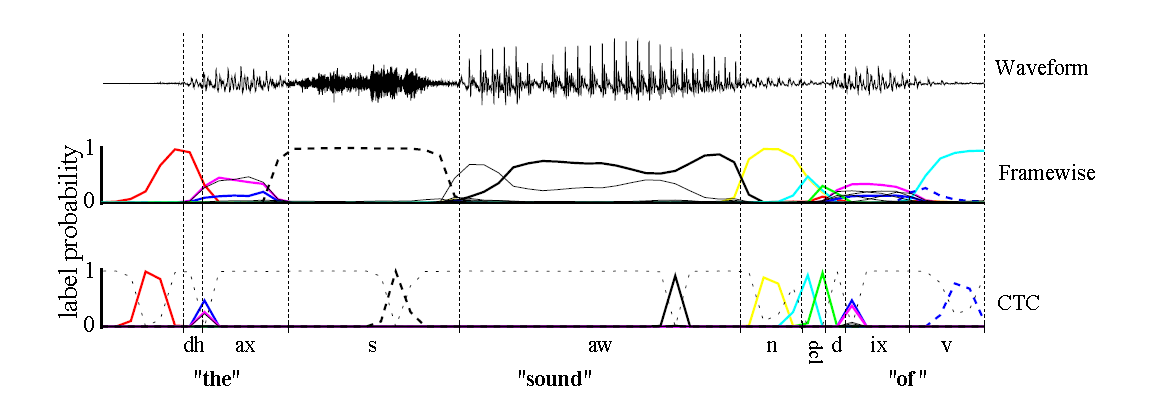如上图，传统的Framewise训练需要进行语音和音素发音的对齐，比如“s”对应的一整段语音的标注都是s；而CTC引入了blank（该帧没有预测值），“s”对应的一整段语音中只有一个spike（尖峰）被认为是s，其他的认为是blank。对于一段语音，CTC最后的输出是spike的序列，不关心每一个音素对应的时间长度。

输出

语音识别中的DNN训练，每一帧都有相应的状态标记，比如有5帧输入x1,x2,x3,x4,x5，对应的标注分别是状态a1,a1,a1,a2,a2。
CTC的不同之处在于输出状态引入了一个blank，输出和label满足如下的等价关系： F(a−ab−)=F(−aa−−abb)=aab<!--//--><![CDATA[//><!--
F(a-ab-)=F(-aa--abb)=aab
//--><!]]>
多个输出序列可以映射到一个输出。

参考

《Supervised Sequence Labelling with Recurrent Neural Networks》 chapter7`
展开全文神经网络
• ## CTC原理介绍

千次阅读 2019-01-13 15:33:54
1. 引言  现实生活当中，序列标注任务随手...这样一来，就会导致数据集的构造特别麻烦，需要耗费大量的人力和时间，因此，CTC方法的提出就是为了解决这个问题，该方法可以不用要求对输出的标签进行对齐，直接利用R...
• ## CTC Loss和Focal CTC Loss

千次阅读 2019-07-24 17:48:27
最近一直在做手写体识别的工作，其中有个很重要的loss那就是ctc loss，之前在文档识别与分析课程中学习过，但是时间久远，早已忘得一干二净，现在重新整理记录下 本文大量引用了- CTC Algorithm Explained Part 1：...
• ## CTC

千次阅读 2019-06-03 17:30:17
聊到CTC（Connectionist Temporal Classification），很多人的第一反应是ctc擅长单行验证码识别： ctc可以提高单行文本识别鲁棒性（不同长度不同位置 ）。今天David 9分享的这篇文章用几个重点直观的见解把ctc讲...
• 这里写自定义目录标题欢迎使用Markdown编辑器新的改变功能快捷键合理的创建标题，有助于目录的生成如何改变文本的样式插入链接与图片如何插入一段漂亮的代码片生成一个适合你的列表创建一个表格设定内容居中、居左、...语音识别
• ## CTC 讲解

千次阅读 2018-06-06 12:44:59
Connectionist Temporal Classificationhttps://sunnycat2013.gitbooks.io/blogs/content/posts/ctc/learning-ctc.html因为最近做了...在学习 CTC 的时候，也看了不少博客，但是我觉得讲的最好的还是原论文 Connect...
• 一、CTC是什么？ CTC可以生成一个损失函数，用于在序列数据上进行监督式学习，不需要对齐输入数据及标签，经常连接在一个RNN网络的末端，训练端到端的语音和文本识别系统。(一句话总结就是当label长度小于input长度...
• 包含CT重建模拟软件CTSim，可以创建模型-生成投影数据-滤波-采用不同重建算法重建及各种优化功能，非常适合初学者。 另含CTSim帮助文档，使用手册，及软件源代码。
• ## 扇形束CT重建算法

热门讨论 2011-10-13 13:35:08
扇形束CT成像几何中，滤波反投影算法，Matlab版本。
• CT重建的原理和现状 CT重建的原理和现状 深度重建：基于深度学习的图像重建 深度重建：基于深度学习的图像重建
• 基本CT测量值是横截面内有效线性衰减系数的线积分。 但线积分不是我们想要的; 我们真的想在整个横截面上得到μ的图片，或等效的CT编号h。 因此，重要的问题是：我们能否在给出其线积分的集合的情况下重建μ的图像？ ...
• CT 医学图像重建 算法 c++实现。CT 医学图像重建 算法 c++实现